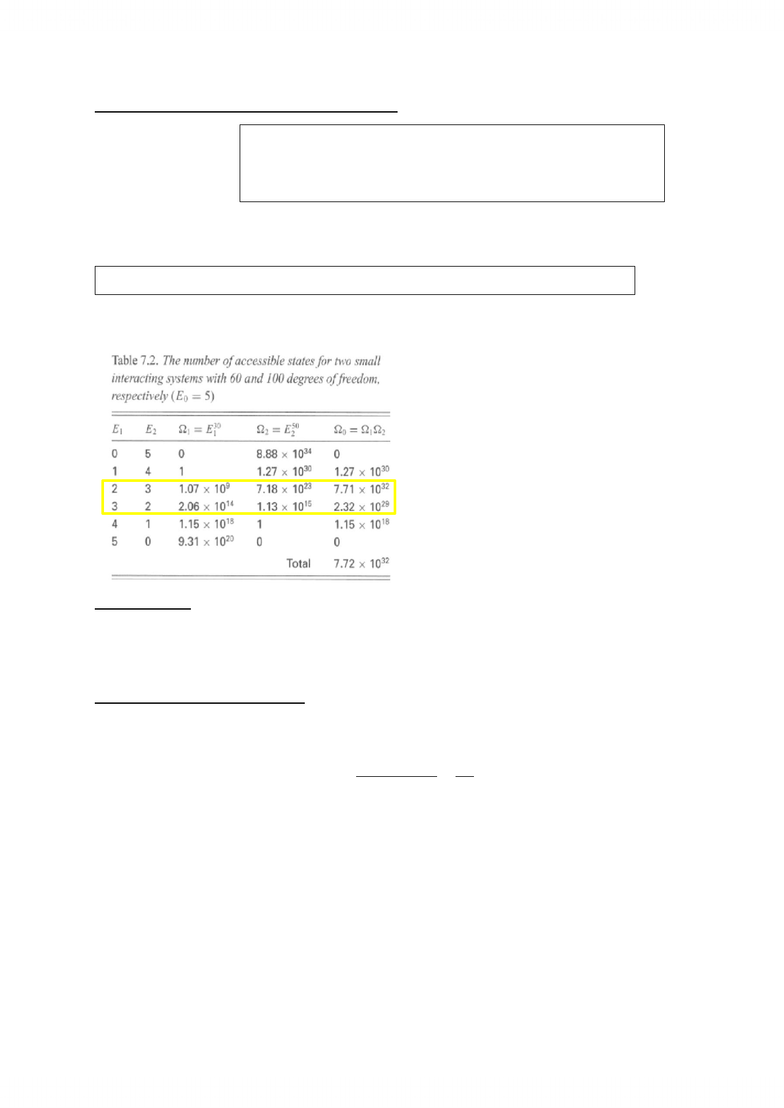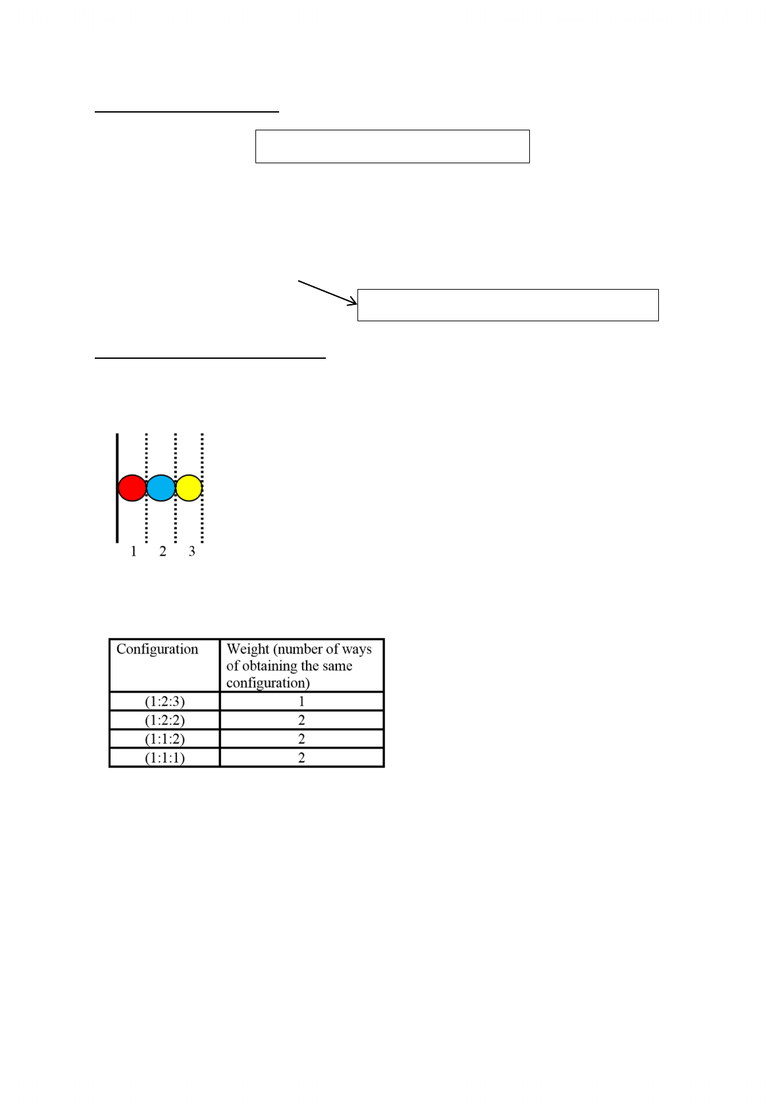Get 2 days of unlimited access
Study Guides (350,000)
NZ (70)
MU (5)
123 (3)

# 123.201 Study Guide - Quotient Rule, Lagrange Multiplier, Weighted Arithmetic Mean

Department
Chemistry
Course Code
123.201
Professor
John Harrison

This preview shows pages 1-3. to view the full 9 pages of the document.123201 Notes
1
Statistical Mechanics
relating the thermodynamic properties to behaviour at an atomic level
The Quantum Structure of Matter
There are a variety of ways that a molecule can store energy:
Translational
Rotational
Vibrational
Electrical
The Quantum States are the certain values that are allowed for each type of energy (as all
the types of energy above are quantised)
 

Differential:  
The total energy can be altered by either changing the population in each level (dni) or
changing the energy of the levels themselves (dεi) often it is hard to change the energy
levels, so the population of each energy level is changed
The entropy is a measure of the spread of the occupied levels
Probability and Entropy
Basic Probability Concepts
Independent Events occur when the outcomes in each event does not depend on the
outcome of any other events.
Mutually Exclusive Events is when one outcome prevents that value from being obtained as
an outcome of any of the other events.
Multiplicity of Events is a weighting for each type of event. There can be multiple ways of
the same outcome occurring.
Correlated Events is when the outcome of one event influences the outcome of another
εi = the energy of quantum state i
ni = the number of molecules in quantum
state i
nA = represent the number in the category A
N = total number of possible outcomes

Only pages 1-3 are available for preview. Some parts have been intentionally blurred.123201 Notes
2
Relationship between Probability and Entropy
 
        
A maximum for S is equivalent to the most probable state (and largest W)
There can be a significant different
between the distribution of energy in
the most probable state and the next
probable.
In this example there is more than 500
ways of distributing the energy in the
most probable state.
Lattice Models
take advantage of the quantum mechanical nature of matter, where not all values
for all quantities are possible
A Lattice Model for Gas Pressure
Arranging N objects into M sites:
  
 
The probability that the molecules are concentrated in one area is very unlikely, with the
most probable arrangement that sites are filled over the whole space.
S = Entropy
k = Boltzmann’s Constant
W = probability of finding the molecules in a particular configuration
or microstates
R = ideal gas constant and NA = Avogadro’s Number

Only pages 1-3 are available for preview. Some parts have been intentionally blurred.123201 Notes
3
A Lattice Model for Diffusion
  
The most even distribution of particles corresponds to the largest number of
possible permuations
The system will evolve towards the state of maximum multiplicity (most even
distribution of particles)
A Lattice Model for a Polymer Chain
This is used for predicting the polymer behaviour when it is fixed at one end to a surface
In this example the red polymer unit remains fixed to the surface. The
possible configurations (R:B:Y) are:
(1:2:3), (1:2:2), (1:1:2), (1:1:1)
However not all the configurations have the same weight, multiple ways of arranging the
units can arise in certain configurations
The average distance from the surface
polymer is equal to the weighted average of
the furthest distance:
Average Length = (1x3 +2x2+2x2+2x1)/7 =
1.86
This proves that the most likely state for a polymer is the partially contracted state (this is a
statistical interpretation of elasticity).
W = number of permutation possibilities
relates to the Second Law of Thermodynamics
###### You're Reading a Preview

Unlock to view full version